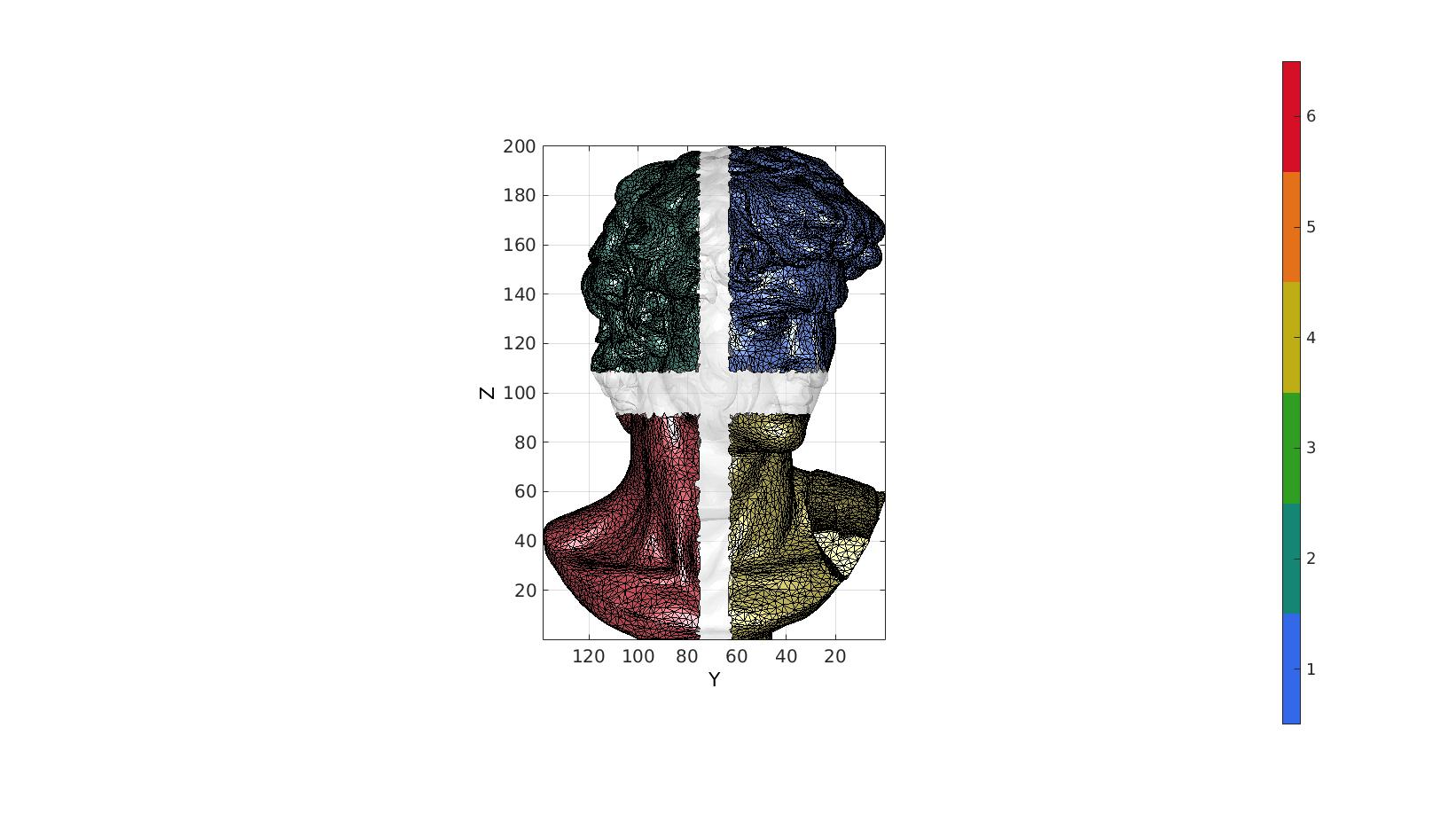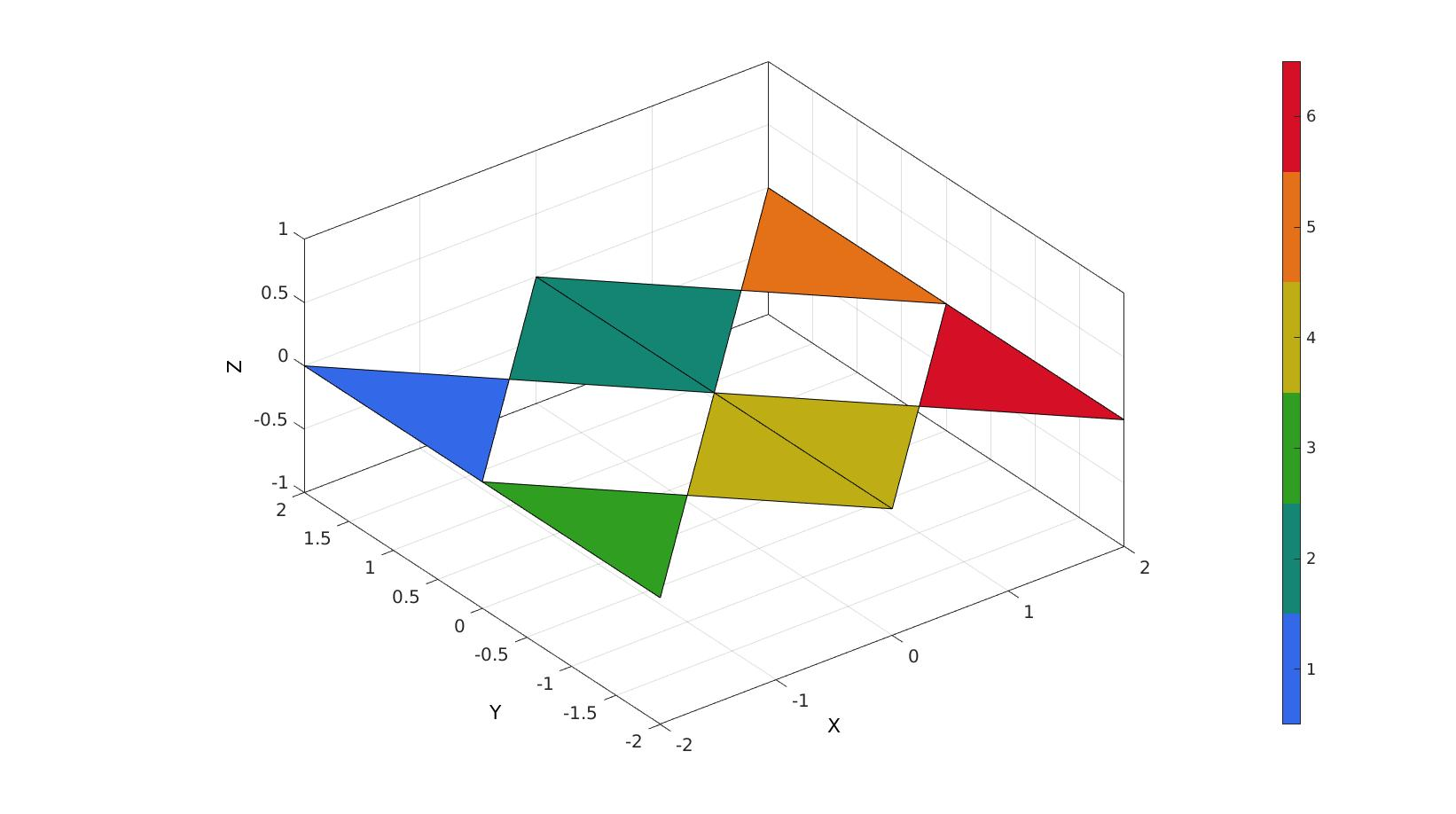# tesgroup

Below is a demonstration of the features of the tesgroup function

## Contents

```clear; close all; clc;
```

## Syntax

[G,G_iter]=tesgroup(F,indExclude);

## Example: grouping detached mesh components

```% Simulating a mesh consisting of seperate pieces.

[F,V]=graphicsModels(9);

%Cut mesh in different directions
logicRemoveVertices=false(size(V,1),1);
for q=2:3
w=max(V(:,q))-min(V(:,q));
m=0.5*(max(V(:,q))+min(V(:,q)));
logicRemoveVertices=logicRemoveVertices | ((V(:,q)>=(m-w/25)) & V(:,q)<=(m+w/25));
end
logicKeep=all(~logicRemoveVertices(F),2);

% Creating detached mesh set
[Fs,Vs]=patchCleanUnused(F(logicKeep,:),V);
```

Grouping mesh components

```optionStruct.outputType='label';
G=tesgroup(Fs,optionStruct);
```

Visualize grouped mesh

```cFigure;
gpatch(F,V,'w','none',0.3);
gpatch(Fs,Vs,G,'k',1);
axisGeom;
view([-90, 0]);
colormap gjet; icolorbar;
drawnow;
```## Example: grouping while avoiding bowtie problem

Create example bowtie problem

```V=[-1 1 0; -1 -1 0; 0 0 0; 1 -1 0; 1 1 0];
F=[1 2 3; 3 4 5];

V(:,2)=V(:,2)+1;
V2=V;
V2(:,2)=-V2(:,2);
V=[V;V2];
F=[F; F+size(V2,1)];

V(:,1)=V(:,1)-max(V(:,1));
V2=V;
V2(:,1)=-V2(:,1);
V=[V;V2];
F=[F; F+size(V2,1)];

[F,V]=mergeVertices(F,V);

% Sub-triangulate to test different densities
% [F,V]=subtri(F,V,3);

Eb=patchBoundary(F,V);
```
```% Get patch connectivity
C=patchConnectivity(F,V);
E=C.edge.vertex;
vertexEdgeConnectivity=C.vertex.edge;

% Work out membership to boundary set
sizVirt=size(V,1)*ones(1,2);
ind_uniqueEdges=sub2indn(sizVirt,sort(E,2));
ind_boundaryEdges=sub2indn(sizVirt,sort(Eb,2));
logicIsBoundaryVertex=ismember(ind_uniqueEdges,ind_boundaryEdges);

vertexBoundaryEdgeConnectivity=vertexEdgeConnectivity;
vertexBoundaryEdgeConnectivity(vertexBoundaryEdgeConnectivity>0)=logicIsBoundaryVertex(vertexBoundaryEdgeConnectivity(vertexBoundaryEdgeConnectivity>0));

indBoundaryVertices=unique(Eb(:));

logicVertexBowtied=sum(vertexBoundaryEdgeConnectivity,2)>2;

indExclude=find(logicVertexBowtied);
```
```clear optionStruct;
optionStruct.indExclude=indExclude;

[G,G_iter]=tesgroup(F,optionStruct);
[I,J]=find(G);
C=double(G);
C(G)=J;
C=max(C,[],2);
```
```cFigure; hold on;
gpatch(F,V,C);

axisGeom;
colormap gjet; icolorbar;
drawnow;
```GIBBON www.gibboncode.org

Kevin Mattheus Moerman, [email protected]

GIBBON footer text

GIBBON: The Geometry and Image-based Bioengineering add-On. A toolbox for image segmentation, image-based modeling, meshing, and finite element analysis.

Copyright (C) 2019 Kevin Mattheus Moerman

This program is free software: you can redistribute it and/or modify it under the terms of the GNU General Public License as published by the Free Software Foundation, either version 3 of the License, or (at your option) any later version.

This program is distributed in the hope that it will be useful, but WITHOUT ANY WARRANTY; without even the implied warranty of MERCHANTABILITY or FITNESS FOR A PARTICULAR PURPOSE. See the GNU General Public License for more details.

You should have received a copy of the GNU General Public License along with this program. If not, see http://www.gnu.org/licenses/.